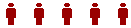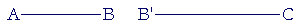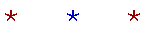An Approach

t o

C A L C U L U S

1

# CONTINUOUS VERSUS DISCRETE

Common boundary

THE SUBJECT MATTER OF DIFFERENTIAL CALCULUS is rate of change; specifically, the rate of change at a given value of a continuous function, such as the speed of motion. What was the speed at exactly 5 seconds after 0? We therefore begin by distinguishing what is continuous from what is discrete.

A natural number is a collection of indivisible and separate units.The people in the room, the electrons in an atom, the names of numbers.  They are discrete units. You cannot take half of any one. If you do, it will not be that unit -- it will not have that same name -- any more. Half of what is called a person is not also a person.

We count things that are discrete:  One person, two, three, four, and so on.

But consider the distance between A and B.  That distance is notcomposed of discrete units.  There is nothing to count -- it is not a number of anything.  We say, instead, that it is a continuous whole.  That means that as we go from A to B, the line "continues" without a break.

The mathematical line is abstracted from the boundary of a plane figure: the boundary of a circle, a square, and so on. All unbroken lines, curved or straight, are continuous.

Now, a collection of discrete units will have only certain parts.  Of 10 people, we can take only half of them, a fifth, or a tenth.  When we divide any discrete collection, we will eventually come to an indivisible one; in this case, one person.

But since the length AB is continuous, we could divide it into any number of parts.  Not only could we take half of it, we could take any part we please -- a tenth, a hundredth, or a billionth -- because AB is not composed of indivisible units. And most important, any part of AB, however small, will still be a length.

That is the idea of a continuum. There is no limit to the smallness of the parts into which it could be divided. We imagine a continuum to be "infinitely divisible," which is a brief way of saying that no matter into how many parts it has been divided, it could be divided still further. And each part will itself be infinitely divisible.

Common boundary

A defining property of a continuous quantity, such as the line AC,is that if it is divided at any point B, then the right-hand boundary B of the part AB, coincides with the left-hand boundary B of the part BC. The parts AB, BC make contact -- they are connected -- at the point B. That allows AB to continue into BC without a gap.

In other words, if a continuous quantity were decomposed into partsor intervals, then all parts are connected.  All parts share their boundaries.The lines AB, B'C do not share a common boundary, a common endpoint -- they are not connected.  And so there is not a continuous line that joins A and C.

But if we join BB', then what were originally two endpoints, twoboundaries, become one.  AB now continues into BC without a gap.

The word continuous comes from a Latin root meaning held together. What is it that holds a line together to make it whole? Again, no matter where a line might be divided, the right and left endpoints, as B above, coincide as one.

In Lesson 3 we will see how that leads to the definition of a continuous function.

Quantities that are continuous we call a magnitude. Magnitudes are of different kinds:  distance, area, time, speed.

In calculus and physics, we regard magnitudes as being measureable. Since they are continuous, we could divide a magnitude into any units of measure, however small. We could divide time, for example, into seconds, or hundredths of a seconds, or trillionths of a second.

We sum this up in the following definition:

DEFINITION 1.  We say that a quantity is continuous if there is no limit to the smallness of the parts into which it could be divided, and 1) no matter where it might be divided, the parts share a common boundary, and 2) each part is a quantity of the same kind.

See the Problem below.

*

The prime example of a continuum is length. A line could be divided into any number of parts, which themselves could be divided; the line will then be composed of those parts. A continuum cannot be composed of points, because points are indivisible; they cannot be divided into parts, as required by the definition. "Point" is a convenient word, when we need it, to refer to the boundary of an interval or where two lines meet. But points do not exist until we point to them! In calculus, anything more than that is unnecessary. In fact, if points were in any sense real entities, then the "two" points, B, B' above, could not become one.

That, at any rate, has been the meaning of the words point and continuum since ancient times. In the 19th century, the abstractions of modernism found their expression in mathematics as well, and certain mathematicians created a radically different meaning for those words. They began with what they called "points," and they ascribed to them a primary logical existence. They then defined a "continuum," and specifically a "line," as a "set" of those "points." (That meaning of  "point" became unexplainedly linked with the geometrical meaning.) And what were called the real "numbers" were then identified with the infinity of those "points." That is logic, which does not require that words have their customary meanings -- or any meaning for that matter. It requires only that words -- "point," "number," "infinity" -- obey the formal rules of a language. A logical theory therefore may be nothing more than a formal game, even to the point of fantasy.

See the Appendix: Are the real numbers really numbers?

Problem.   Which of these is continuous and which is discrete?

Do the problem yourself first!

a)  The leaves on a tree.   Discrete

b)  The stars in the sky.   Discrete

c)  The distance from here to the Moon.  Continuous. Our idea of

distance, of length, is that it could have any size, however large or however small.

d)  A bag of apples.   Discrete

e)  Applesauce.   Continuous!

f)  A dozen eggs.   Discrete. (But if they're scrambled?)

g)  60 minutes.   Continuous. Our idea of time, like our idea of

distance, is that there is no smallest unit. Any part of 60 minutes is still time.

h)  Motion from one place to another.   Continuous. The idea of any

quantity of motion is that there is no limit to its smallness.

i)  Pearls on a necklace   Discrete

j)  The area of a circle.

As area, it is continuous; any part of an area is also an area. But as a form, a circle is discrete; half a circle is not also a circle.

k)  The volume of a sphere.

As volume, it is continuous. As a form, a sphere is discrete.

l)  A gallon of water.

Continuous. We think of volume as having any part. And any part is still a volume of water.

But:

m)  Molecules of water.

Discrete. In other words, if we could keep dividing a quantity of water, then ultimately (in theory) we would come to one molecule. If we divided that, it would no longer be water!

n)  A chapter in a book.

Discrete. Surely, half a chapter is not also a chapter.

o)  Events.

If you think that half an event is also an event, then you will say that an event -- such as a birthday party -- is continuous. (We are not speaking of the time in which the event occurs. We are speaking of the event itself.) Otherwise, you will say that events are discrete.

p)  The changing shape of a balloon as it's being inflated.

Continuous. The shape is changing continuously.

q)  The evolution of biological forms; that is, from fish to man
n)  (according to the theory).

What do you think? Was it like a balloon being inflated? Or was each new form discrete?

r)  Words.  Discrete.

s)  Ideas.

If you think that the hundredth part of an idea is also an idea, (Really?), then you will say that ideas are continuous.

t)  Meanings.

Discrete. Half a meaning?

u)  The proof of a theorem.

Discrete.  Half a proof?

v)  The names of numbers.

Surely, the names of anything are discrete.

w)  The universe.

Discrete.  Is half a universe also a universe?

Apart from our conceptions of time, space, and motion, we see that virtually everything we encounter is discrete.  Even a motion picture -- where the figures on the screen appear to be in continuous motion -- is made up of individual frames, which are discrete.

Calculus, however, is the study of magnitudes; of things that are continuous.Next Lesson:  Limits

Please make a donation to keep TheMathPage online.
Even \$1 will help.